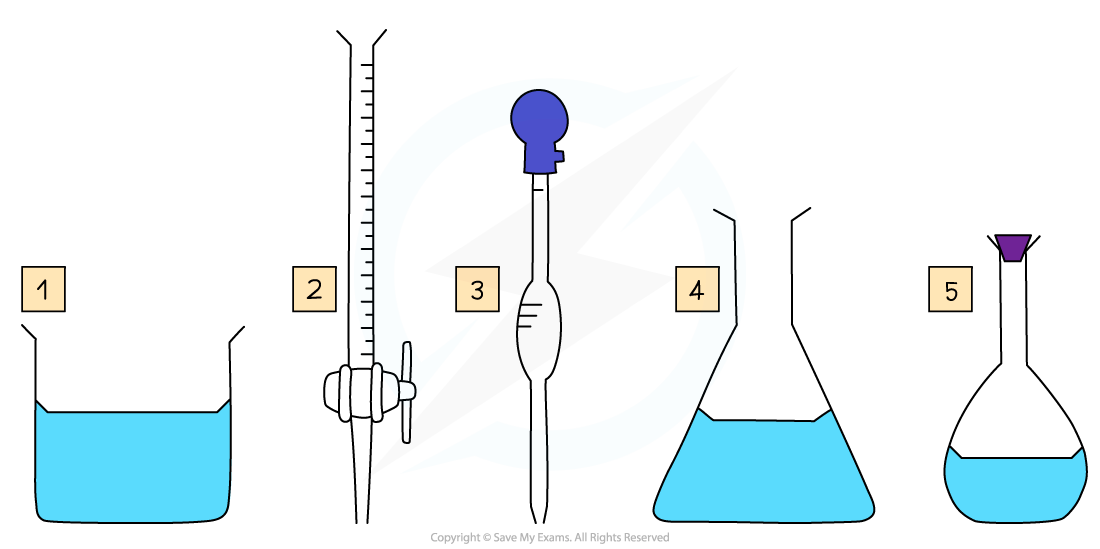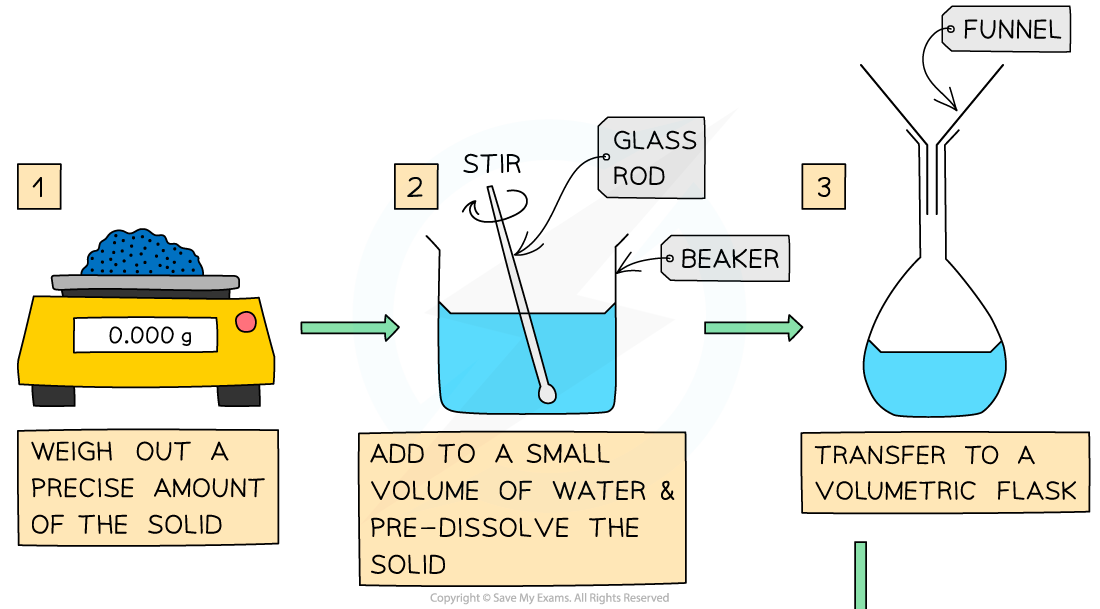# AQA A Level Chemistry复习笔记4.1.1 Making a Volumetric Solution

### Making a Volumetric Solution

#### Volumetric Analysis

• Volumetric analysis is a process that uses the volume and concentration of one chemical reactant (a volumetric solution) to determine the concentration of another unknown solution
• The technique most commonly used is a titration
• The volumes are measured using two precise pieces of equipment, a volumetric or graduated pipette and a burette
• Before the titration can be done, the standard solution must be prepared
• Specific apparatus must be used both when preparing the standard solution and when completing the titration, to ensure that volumes are measured preciselySome key pieces of apparatus used to prepare a volumetric solution and perform a simple titration

1. Beaker
2. Burette
3. Volumetric Pipette

#### Making a Volumetric Solution

• Chemists routinely prepare solutions needed for analysis, whose concentrations are known precisely
• These solutions are termed volumetric solutions or standard solutions
• They are made as accurately and precisely as possible using three decimal place balances and volumetric flasks to reduce the impact of measurement uncertainties
• The steps are:#### Volumes & concentrations of solutions

• The concentration of a solution is the amount of solute dissolved in a solvent to make 1 dm3 of  solution
• The solute is the substance that dissolves in a solvent to form a solution
• The solvent is often water

• A concentrated solution is a solution that has a high concentration of solute
• A dilute solution is a solution with a low concentration of solute
• Concentration is usually expressed in one of three ways:
• moles per unit volume
• mass per unit volume
• parts per million

#### Worked Example

Calculate the mass of sodium hydroxide, NaOH, required to prepare 250 cm3 of a 0.200 mol dm-3 solution

Step 1: Find the number of moles of NaOH needed from the concentration and volume:

number of moles  = concentration (mol dm-3) x volume (dm3)

n = 0.200 mol dm-3 x 0.250 dm3

n = 0.0500 mol

Step 2: Find the molar mass of NaOH

M = 22.99 + 16.00 + 1.01 = 40.00 g mol-1

Step 3: Calculate the mass required

mass = moles x molar mass

mass =  0.0500 mol x 40.00 g mol-1   = 2.00 g